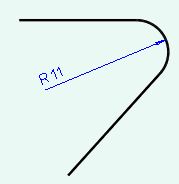> > > Modify 2D geometry using dimensions (3D annotations)

Modify 2D geometry using dimensions (3D annotations)
Creo Elements/Direct Modeling allows you to modify 2D geometry using dimensions. You can select dimensions and then use modeling commands to modify the 2D geometry. Such dimensions are called driving dimensions. The dimensions that can be used as driving dimensions are listed in this topic.
To modify a 2D geometrical element using a dimension,
1. Select a 2D linear, angular, radial, or diameter dimension in the viewport. The visual feedback shows the 2D CoPilot arrow (dragger), which you can drag to modify the 2D geometrical element that is connected to the dimension. The Command Mini Toolbar (CMT) opens.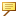If you select a radial or a diameter dimension in the viewport, the Change Radius or the Change Diameter command starts by default.
For linear dimensions:
Select a point on the right half of the dimension to modify the line, which is to the right of the dimension.Select the a point on the left half of the dimension to modify the line, which is to the left of the dimension.For angular dimensions: The 2D geometrical elements that are modified depend on the selection of the angular dimension. For example, select a point on the angular dimension that is closer to one line to modify that line.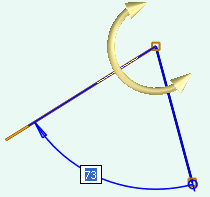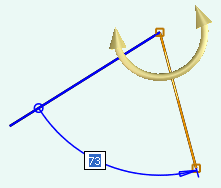2. You can either drag the 2D CoPilot or type a value to modify the 2D geometry. You can also select an alternative command on the CMT, and then drag the 2D CoPilot to modify the 2D geometry. See Using the Command Mini Toolbar (CMT) for details.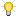• After you select a dimension, the default command depends on the type of the dimension and the geometrical element to which the dimension is connected.• If the 3D Documentation module is active, the CMT shows 3D Documentation commands.• You can also move or delete the selected dimension using the CMT.
3. Click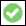to complete the operation.
Limitations
You cannot use the following dimensions as driving dimensions to modify 2D geometry:
Dimensions created on a fillet edge.